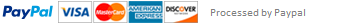### Change Calculator in Python

In this project you will be programming toward a solution of a problem using decision making blocks such as if statements. Your project must contain the use of if, else, elif blocks.

You will write a program that will prompt the user for the total change amount as an integer input, and your program will output the change using the fewest coins, one coin type per line.

The coin types are dollars, quarters, dimes, nickels, and pennies.

Use singular and plural coin names as appropriate, like 1 penny vs. 2 pennies.

Example of a sample run:

Enter the change amount as integer:123

1 dollar

2 dimes

3 pennies

Enter the change amount as integer:45

1 quarter

2 dimes

Enter the change amount as integer:-45

No Change

Enter the change amount as integer:100

1 dollar

Enter the change amount as integer:125

1 dollar

1 quarter

LAB ACTIVITY

23.1.1:: Finding the Exact change using Decision-Making blocks

print("This program asks the user to enter a change amount using integers only,")

print("and outputs the change using the fewest coins.")

input_val = int(input("Enter the change amount as integer:"))

''' Type your code here. '''

This assignment has been answered 2 times in private sessions.